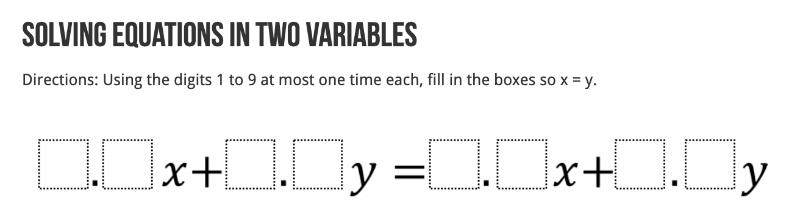# Open Middle: Solving Equations in Two VariablesCreation of this problem was inspired by this OpenMiddle problem submitted by Daniel Luevanos. Teachers: You can create different variants of this problem by simply entering a different Goal Equation. Here, the goal equation is (dashed line). Yet this can be changed.

## Fill in the input boxes (up top) using digits 1-9 so that no digit repeats. Can you create the equation y = x (dashed line)?

Explain or show your thought process as to how you arrived at your solution. How can you algebraically show your solution is correct?

## Try to find another solution (different from the one you found above).

Explain or show your thought process as to how you arrived at your solution. How can you algebraically show your solution is correct?